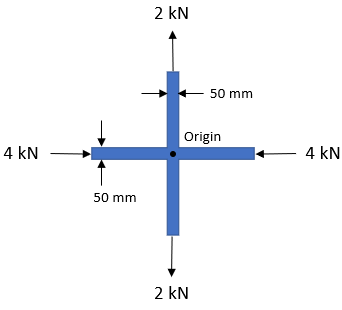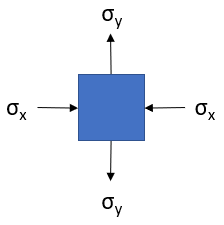## Cross Bar

In the below diagram, a cross plane member has a 15 mm uniform thickness and some loads in compression/tension. What is the max shear stress at the origin?Hint
Stress:
$$\sigma = \frac{Load}{Area}$$$Hint 2 The max shear stress considering three dimensions: $$\tau_{max}=\frac{\sigma_y-\sigma_x}{2}$$$
The stress at the origin can be represented as:which looks like a typical Mohr's Circle problem. To construct a Mohr's circle, the following sign conventions are used:

Since $$stress=\frac{load}{area}$$
$$\sigma_x=\frac{4,000N}{(0.05m)(0.015m)}=-5.33\:MPa$$$$$\sigma_y=\frac{2,000N}{(0.05m)(0.015m)}=2.67\:MPa$$$
Since $$\sigma_x$$ is compressive:
$$\sigma_1=2.67\:MPa$$$$$\sigma_2=0\:MPa$$$
$$\sigma_3=-5.33\:MPa$$$The max shear stress considering three dimensions is always: $$\tau_{max}=\frac{\sigma_1-\sigma_3}{2}=\frac{2.67-(-5.33)}{2}=4\:MPa$$$
4 MPa